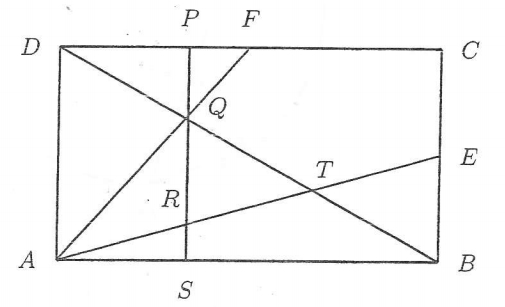# Find the area...

Geometry Level 3The diagram above shows a rectangle $ABCD$ such that $E$ is the midpoint of $BC$ and $F$ is the midpoint of $CD$. The diagonal $BD$ intersects $AF$ and $AE$ at $Q$ and $T$ respectively. The vertical line $PS$ passing through $Q$ is perpendicular to $AB$ and intersects $AE$ at $R$. It is also given that $AB=CD=12$ and $BC=AD=6$.

Find the area of the triangle $\triangle QRT$.

×